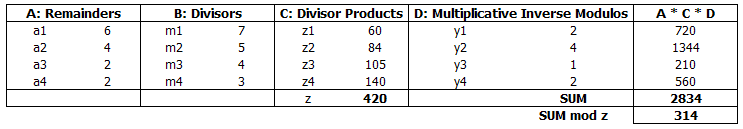# Using Chinese Remainder Theorem IRL

In 1999 I had the privilege of attending the KRMO (Karnataka Regional Maths Olympiad) camp at IISc. While it didn’t do much to improve my math capabilities, it taught me a lot about how to preserve self-esteem when in the presence of materially smarter, sharper & more capable folks.

One of the quaint bits of math that I did learn was the Chinese Remainder Theorem and true to its name it has remained in the recesses of my brain since unused as yet another thing I learnt in school which I would never use.

Until today.

Earlier today I uploaded a large number of files into Google Drive in batches of 300 or so, a process that took close to an hour. When the upload was done Google helpfully let me know that some uploads in my last batch had failed.

a. It creates duplicates of files when you re-upload without checking for conflicts

b. It doesn’t tell you the number of files that exist in a folder

I needed a quick way of figuring out how many files I was missing. Since only the last batch failed it was between 0 and 300.

Exasperated I listed contents and started counting the number of files manually. Not surprisingly I quickly lost count. I switched to grid mode and scrolled right down when I saw this:I stretched out the window and saw this:I had an epiphany - this was a job for CRT.

I quickly wrote down the number of files in the last row for different column sizes like this:

**Number of Columns &nbsp; Number of Files displayed in last row** 7 6
6 2
5 4
4 2
3 2


The number of files in the last row of each grid represents the remainder when N, the number of files in the folder, is divided by the number of columns being displayed. The question for CRT is to compute the total number of files N in the folder based on these remainders. This looks almost impossible to someone who hasn’t seen CRT at work.

After a few minutes of googling I found the method for reconstructing N here:

http://homepages.math.uic.edu/~leon/mcs425-s08/handouts/chinese_remainder.pdf

The math works out like so:

1. Drop the 6 column case because we need co-prime divisors
2. Compute the product of the co-prime divisors = z
3. Compute z1..z4 as z/m1…z/m4
4. Compute multiplicative inverse modulos y1..y4 (this takes a slight amount of effort)
5. Compute SumProduct of Remainders, Divisor Products and MIMs
6. Compute remainder R as the SumProduct modulo zCRT now tells us that N must be of the form k*z + R where k is an integer > 0

k=1 => 420+314 = 714
k=2 => 840+314 = 1154
k=3 => 1260+314 = 1574
k=4 => 1680+314 = 1994


I was looking to upload 1579 files and I had transferred at least 1200 until the last batch so 1574 was the number I was after. I was missing just 5 files.

 Of course, this hardly solves the problem - I still need to find those 5 and re-upload them. :